## Great Circle

The shortest path between two points on a Sphere, also known as an Orthodrome. To find the great circle (Geodesic) distance between two points located at Latitudeand Longitudeofand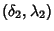on a Sphere of Radius, convert Spherical Coordinates to Cartesian Coordinates using(1)

(Note that the Latitudeis related to the Colatitudeof Spherical Coordinates by, so the conversion to Cartesian Coordinates replaces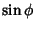andby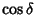and, respectively.) Now find the Anglebetweenandusing the Dot Product,(2)

The great circle distance is then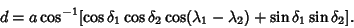(3)

For the Earth,the equatorial Radius iskm, or 3963 (statute) miles.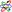Unfortunately, the Flattening of the Earth cannot be taken into account in this simple derivation, since the problem is considerably more complicated for a Spheroid or Ellipsoid (each of which has a Radius which is a function of Latitude).

The equation of the great circle can be explicitly computed using the Geodesic formalism. Writing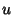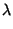(4)(5)

gives the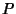,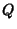, andparameters of the Geodesic (which are just combinations of the Partial Derivatives) as(6)(7)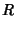(8)

The Geodesic differential equation then becomes(9)

However, because this is a special case ofwithandexplicit functions ofonly, the Geodesic solution takes on the special form(10)

(Gradshteyn and Ryzhik 1979, p. 174, eqn. 2.599.6), which can be rewritten as(11)

It therefore follows that(12)

This equation can be written in terms of the Cartesian Coordinates as(13)

which is simply a Plane passing through the center of the Sphere and the two points on the surface of the Sphere.

See also Geodesic, Great Sphere, Loxodrome, Mikusinski's Problem, Orthodrome, Point-Point Distance--2-D, Sphere

References

Gradshteyn, I. S. and Ryzhik, I. M. Tables of Integrals, Series, and Products, 5th ed. San Diego, CA: Academic Press, 1979.

Weinstock, R. Calculus of Variations, with Applications to Physics and Engineering. New York: Dover, pp. 26-28 and 62-63, 1974.